PEAK-Rules/Syntax-Matching UserPreferences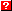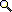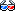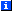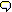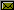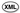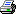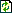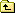# Matching Python Syntax

The peak.rules.syntax module allows you to define pattern-matching predicates against snippets of parameterized Python code, such that a rule expression like:

```syntax.match(expr, type(`x`) is `y`) and y in Const
```

Will return true if expr is a PEAK-Rules AST of the form:

```Compare(Call(Const(type), (v1,)), ('is', Const(v2)))
```

(where v1 and v2 are arbitrary values).

# Bind Variables

Bind variables are placeholders in a pattern that "bind" themselves to the value found in that location in the matched data structure. Thus, in the example above, `x` and `y` are bind variables, and cause "y" in the later part of the expression to refer to the right-hand side of the is operator being matched. (The arbitrary value v2 in the example above.)

Bind variables are represented within a tree as an AST node created from the variable name:

```>>> from peak.rules.syntax import Bind

>>> Bind('x')
Bind('x')
```

# Compiling Tree-Match Predicates

The match_predicate(pattern, expr, binds) function is used to combine a pattern AST and an expression AST to create a PEAK-Rules predicate object that will match the specified pattern. The binds argument is a dictionary mapping from bind-variable names to lists of expression ASTs, and is modified in-place as the predicate is assembled:

```>>> from peak.rules.syntax import match_predicate
```

Rules defined for this function will determine what to do based on the type of pattern. If pattern is a bind variable, the binds dictionary is updated in-place, inserting expr under the bind variable's name, and True is returned, indicating that this part of the pattern will always match:

```>>> from peak.util.assembler import Local
>>> b = {}

>>> match_predicate(Bind('x'), Local('y'), b)
True

>>> b
{'x': [Local('y')]}
```

If there's already an entry for that variable in the binds dictionary, a more complex predicate is returned, performing an equality comparison between the new binding and the old binding of the variable, and the value in binds is updated:

```>>> match_predicate(Bind('x'), Local('z'), b)
Test(Truth(Compare(Local('z'), (('==', Local('y')),))), True)
```

This is so that patterns like "x is not x" will actually compare the two "x"s and see if they're equal. Of course, if you bind the same variable more than once to equal expression ASTs, you will not get back a comparison, and the binds will be unchanged:

```>>> match_predicate(Bind('x'), Local('z'), b)
True

>>> b
{'x': [Local('y'), Local('z')]}
```

Finally, there is a special exception for bind variables named `_`: that is, a single underscore. Bind variables of this name are never stored in the binds, and always return True as a predicate, allowing you to use them as "don't care" placeholders:

```>>> any = Bind('_')
>>> match_predicate(any, Local('q'), b)
True

>>> b
{'x': [Local('y'), Local('z')]}
```

## Matching Structures and AST nodes

For most node types other than Bind, the predicates are a bit more complex. By default, the predicate should be an exact (istype) match of the node type, intersected with a recursive application of match_predicate() to each of the target node's children. For example:

```>>> b = {}
>>> from peak.util.assembler import *
>>> from peak.rules.codegen import *

>>> match_predicate(Add(any, any), Local('q'), b)
Test(IsInstance(Local('q')), istype(<class '...Add'>, True))

>>> b
{}
```

Each child is defined via a Getitem() operation on the target node, so that any placeholders and criteria will target the right part of the tree:

```>>> match_predicate(Add(Bind('x'), Bind('y')), Local('q'), b)
Test(IsInstance(Local('q')), istype(<class '...Add'>, True))

>>> b
{'y': [Getitem(Local('q'), Const(2))],
'x': [Getitem(Local('q'), Const(1))]}
```

Non-node patterns are treated as equality comparisons:

```>>> b = {}
>>> match_predicate(42, Local('q'), b)
Test(Comparison(Local('q')), Value(42, True))

>>> b
{}
```

Except for None, which produces an is None test:

```>>> match_predicate(None, Local('q'), b)
Test(Identity(Local('q')), IsObject(None, True))

>>> b
{}
```

And sequences are matched by comparing their length:

```>>> match_predicate((), Local('q'), b)
Test(Comparison(Call(Const(<... len>), (Local('q'),),...)), Value(0, True))

>>> match_predicate([], Local('q'), b)
Test(Comparison(Call(Const(<... len>), (Local('q'),),...)), Value(0, True))

>>> b
{}
```

And recursively matching their contents:

```>>> match_predicate((Bind('x'), Add(Bind('y'), any)), Local('q'), b)
Signature([Test(Comparison(Call(Const(<... len>), (Local('q'),),...)),
Value(2, True)),
Test(IsInstance(Getitem(Local('q'), Const(1))),
istype(<class '...Add'>, True))])

>>> b
{'y': [Getitem(Getitem(Local('q'), Const(1)), Const(1))],
'x': [Getitem(Local('q'), Const(0))]}
```

# Parsing Syntax Patterns

The syntax.SyntaxBuilder class is used to parse Python expressions into AST patterns suitable for use with match_predicate:

```>>> from peak.rules.syntax import SyntaxBuilder, match
>>> builder = SyntaxBuilder({}, locals(), globals(), __builtins__)
>>> pe = builder.parse
```

It parses backquoted identifiers into Bind nodes:

```>>> pe('type(`x`) is `y`')
Compare(Call(Const(<type 'type'>), (Bind('x'),), (), (), (), True),
(('is', Bind('y')),))
```

And rejects all other use of backquotes:

```>>> pe('`type(x)`')
Traceback (most recent call last):
...
SyntaxError: backquotes may only be used around an indentifier
```

In all other respects, it's essentially the same as codegen.ExprBuilder.

## The match() Pseudo-function

This isn't really a function, but you can use it in a predicate string in order to perform a pattern match on a PEAK-Rules AST. It's mainly intended for use in extending PEAK-Rules to recognize and replace various kinds of subexpression patterns (e.g. by adding rules to predicates.expressionSignature()), but it can of course also be used in any other tools you build atop PEAK-Rules' expression machinery.

In this example, we show it being used to define a rule that will recognize expressions of the form "type(x) is y", where x and y are arbitrary expressions:

```>>> from peak.rules.syntax import match

>>> from peak.rules.predicates import CriteriaBuilder
>>> builder = CriteriaBuilder(
...     {'expr':Local('expr')}, locals(), globals(), __builtins__
... )
>>> pe = builder.parse

>>> pe('match(expr, type(`x`) is `y`)')
Signature([Test(IsInstance(Local('expr')),
istype(<class 'peak.util.assembler.Compare'>, True)),
Test(IsInstance(Getitem(Local('expr'), Const(1))),
istype(<class 'peak.util.assembler.Call'>, True)),
Test(Comparison(Getitem(Getitem(Local('expr'), Const(1)),
Const(1))),
Value(Const(<type 'type'>), True)),
Test(Comparison(Call(Const(<... len>),
(Getitem(Getitem(Local('expr'),
Const(1)), Const(2)),), (),
(), (), True)),
Value(1, True)),
Test(Comparison(Call(Const(<... len>),
(Getitem(Getitem(Local('expr'), Const(1)),
Const(3)),), (), (), (), True)),
Value(0, True)),
Test(Comparison(Call(Const(<... len>),
(Getitem(Getitem(Local('expr'), Const(1)),
Const(4)),), (), (), (), True)),
Value(0, True)),
Test(Comparison(Call(Const(<... len>),
(Getitem(Getitem(Local('expr'), Const(1)),
Const(5)),), (), (), (), True)),
Value(0, True)),
Test(Comparison(Getitem(Getitem(Local('expr'), Const(1)),
Const(6))),
Value(True, True)),
Test(Comparison(Call(Const(<... len>),
(Getitem(Local('expr'), Const(2)),), (),
(), (), True)),
Value(1, True)),
Test(Comparison(Call(Const(<... len>),
(Getitem(Getitem(Local('expr'), Const(2)),
Const(0)),), (), (), (), True)),
Value(2, True)),
Test(Comparison(Getitem(Getitem(Getitem(Local('expr'),
Const(2)), Const(0)),
Const(0))),
Value('is', True))])

>>> builder.bindings
{'y': Getitem(Getitem(Getitem(Local('expr'), Const(2)), Const(0)), Const(1)),
'x': Getitem(Getitem(Getitem(Local('expr'), Const(1)), Const(2)), Const(0))}
```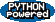EditText of this page (last modified 2010-08-17 19:59:36) FindPage by browsing, title search , text search or an index Or try one of these actions: AttachFile, DeletePage, LikePages, LocalSiteMap, SpellCheck